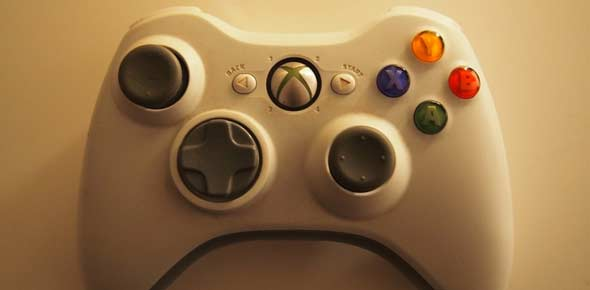# Blitzkrieg (demo)

10 QuestionsSettingsThis is a Demo Test to check the Online Test Environment. Participants can take this Test as many times as they wish.

Related Topics
• 1.
In a class of 9 boys and 7 girls, what are the number of ways of selecting 4 boys and 5 girls?
• A.

2646

• B.

6246

• C.

1260

• D.

2664

• 2.
Two buses start from the opposite points of a main road, 150kms apart. The first bus runs for 25kms and takes a right turn and then runs for 15 kms. It then turns left and runs for another 25 kms and takes the direction back to reach the mai road. In the meantime, due to a minor breakdown, the other bus has run only 35 kms along the main road. What would be the distance between the two buses at this point?
• A.

65kms

• B.

75kms

• C.

80 kms

• D.

85kms

• 3.
In a hospital, the lab technician’s mother’s husband’s father is the attender there whose only child is the ward secretary. How is this child related to the lab technician?
• A.

Mother

• B.

Father

• C.

Son

• D.

None of these

• 4.
The jogging track in a sports complex is 726 metres in circumference. Deepak and his wife start from the same point and walk in opposite directions at 4.5 km/hr and 3.75 km/hr respectively. They will meet for the first time in:
• A.

4.9 min

• B.

5.28 min

• C.

5.5 min

• D.

6 min

• 5.
Imagine that in a calendar year, there were 436 days and 9 day in a week, then how many odd days will be there in that year?
• A.

1

• B.

2

• C.

3

• D.

4

• 6.
Find the missing number : 6,11,21,36,56, …..
• A.

80

• B.

81

• C.

86

• D.

None of these

• 7.
The sum of the squares of three numbers is 138, while the sum of their products taken two at a time is 131. Their sum is:
• A.

20

• B.

30

• C.

40

• D.

None of these

• 8.
A class of 24 boys was divided into two sub-sections containing 12 boys each. What is the probability that the two shortest boys of the class are in different sub-sections, if all boys have different heights?
• A.

7/24

• B.

6/23

• C.

14/23

• D.

12/23

• 9.
In the following the questions choose the word which best expresses the meaning of the given word. RESCUE
• A.

Command

• B.

Help

• C.

Defence

• D.

Safety

• 10.
Choose the pair that best represents a similar relationship to the one expressed in the original pair of words. FERAL : TAME
• A.

Rancid : rational

• B.

Repetitive : recurrent

• C.

Nettlesome : annoying

• D.

Ephemeral : immortal